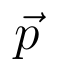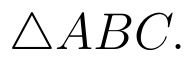Select Page

# 12 Science CBSE Answers for MCQ Maths Differential Equations in English

12 Science CBSE Answers for MCQ Maths Differential Equations in English to enable students to get Answers in a narrative video format for the specific question.

Expert Teacher provides 12 Science CBSE Answers for MCQ Maths Differential Equations through Video Answers in English language. This video solution will be useful for students to understand how to write an answer in exam in order to score more marks. This teacher uses a narrative style for a question from Differential Equations not only to explain the proper method of answering question, but deriving right answer too.

Please find the question below and view the Answer in a narrative video format.

Question:

Answer Video in English:

You can select video Answers from other languages also. Please check Answers in ( Hindi )

## Similar Questions from CBSE, 12th Science, Maths, Differential Equations

Question 1 : Solve the differential equation:(View Answer Video)

Question 2 : Find the sum of the order and the degree of the following differential equation:(View Answer Video)

Question 3 : Write the differential equation formed from the equation y = mx + c, where m and c are arbitrary constants.

Question 4 : Find the particular solution of the differential equationgiven that y = 1, when x = 0. (View Answer Video)

Question 5 : Find the particular solution of the differential equationgiven that y = 1 when x = 0. (View Answer Video)

### Inverse Trigonometric Functions

Question 1 :is equal to : (View Answer Video)

Question 2 : Solve for(View Answer Video)

Question 3 : Solve for(View Answer Video)

Question 4 : Write the principal value of(View Answer Video)

Question 5 : Write the value of the following:(View Answer Video)

### Vector Algebra

Question 1 :  Write the value offor which the vectorsandare parallel vectors.  (View Answer Video)

Question 2 : Find the scalar components of the vectorwith initial point A (2, 1) and terminal point B (-5, 7).  (View Answer Video)

Question 3 : L and M are two points with position vectorsandrespectively. Write the position vectors of a point N which divides the line segment LM in the ratio 2:1 externally.   (View Answer Video)

Question 4 : if a, b, c are unit vectors such that a + b + c = 0, then find the value of a.b + b.c + c.a. (View Answer Video)

Question 5 : The two vectorsandrepresent the two sides AB and AC, respectively ofFind the length of the median